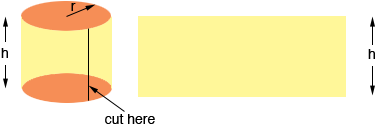SEARCH HOMEMath Central Quandaries & QueriesQuestion from Nina, a student: if a cylinder is 23 ft hih with a 12 ft radius, how do I find the surface area?Hi Nina,

Imagine that the cylinder is made of paper, like the label on a soup can. Suppose it is h units high and the radius is r units. Cut the paper as you would to cut off the label of the soup can, then roll it out to form a rectangle.The rectangle is h units wide. How long is it? What is its area?

PennyMath Central is supported by the University of Regina and The Pacific Institute for the Mathematical Sciences.